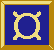## A Simple Pocket Calculator

Using the ideas illustrated by the previous examples you can build a simple pocket calculator.For simplicity, postfix notation is used, i.e., to compute 3+4 you enter 3 Ent 4 +.

Here is the source code:

```import Fudgets

main = fudlogue (shellF "Pocket Calculator" calcF)

calcF = intDispF >==< mapstateF calc  >==< buttonsF

data Buttons = Plus | Minus | Times | Div | Enter | Digit Int   deriving (Eq)

buttonsF = placerF (matrixP 4) (
listF [d 7, d 8, d 9,op Div,
d 4, d 5, d 6,op Times,
d 1, d 2, d 3,op Minus,
hole,d 0,ent, op Plus])
where
d n = (Digit n,buttonF (show n))
ent = op Enter
hole = (Enter,holeF)
op o = (o,buttonF (opLabel o))
where opLabel Plus = "+"
opLabel Minus = "-"
opLabel Times  = "*"
opLabel Div = "/"
opLabel Enter = "Ent"

calc (n:s)   (Digit d,_) = new (n*10+d) s
calc s       (Enter,_)   = (0:s,[])
calc (y:x:s) (Plus,_)    = new (x+y) s
calc (y:x:s) (Minus,_)   = new (x-y) s
calc (y:x:s) (Times,_)   = new (x*y) s
calc (y:x:s) (Div,_)     = new (x `div` y) s
calc s       _           = (s,[])

new n s = (n:s,[n])
```

### Things to note

The program structure is much the same as in the Up/Down/Reset counter.
• To specify the placement of the buttons we have used `placerF` (as in Example 4) and the placer `matrixP` which has the number of columns as an argument.

• The state maintained by the application specific code (the function `calc`) is a stack (represented as a list) of numbers. The function `calc` pushes and pops numbers from the stack as appropriate. The last clause in the definition means that nothing happens if there are too few values on the stack for an operation.

• Previous Example
• Back to the Example list